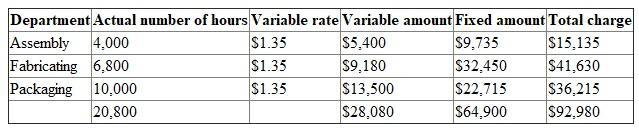# Quiz 7: Allocating Costs of Support Departments and Joint Products

Business

1)Single Charging Rate: Single charging rate method is used in helping the company to allocate the supporting department costs to the other departments. The rate is allocated for better performance evaluation and also to determine the costs of units produced. If a single charge rate is used, fixed costs and variable costs are combined and then divided by estimating activity.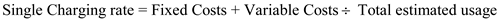Given: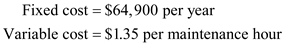Calculate the total expected costs as follows: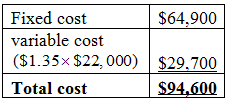Calculate the single charging rate as follows:Therefore, Single charging rate is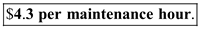2.Calculate the total amount charged for maintenance for the year as follows: The charge based on actual usage is,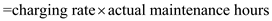Assembly department charge is calculated as follows:Fabricating department charge is calculated as follows:Packaging department charge is calculated as follows:Total amount charged is calculated as follows:Therefore, total amount charged for maintenance is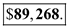3.If the assembly department used 4,000 maintenance hours, then the charge of the three departments would be: Assembly department charge is calculated as follows:Fabricating department charge is calculated as follows:Packaging department charge is calculated as follows: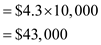Total amount charged is calculated as follows:Explanation: In a company where there are producing departments and the supporting departments there's a problem of allocation of cost of service departments to the producing departments arises. The allocation is necessary to predict the actual cost per unit which is in turn useful in quoting the competent price of the product. • The cost to the producing department is directly attributed to the products or services because that department is directly responsible for manufacturing the products and sale of the products. • The support departments are the departments who support through services to the producing departments in manufacturing or sale of the products. The cost incurred by the support departments are the indirect cost to the production of products by the company. • The examples of supporting departments in the factory are personnel department, payroll department, purchasing department, power department, accounting department, maintenance department etc. All these departments are useful in supporting the production departments and for smooth running of the production. • The cost of the supporting departments is allocated to the producing departments on the basis of cost drivers. The cost of the producing departments is the direct cost to the product. • In the traditional manufacturing environment the two stage allocation process which is used for assigning support service cost to products is as under:- • In the first stage the company identifies the cost incurred individually in every supporting departments (like personnel department, payroll department etc.) and then the cost of the supporting departments will be allocated to the production departments on the basis of cost drivers. • The cost drivers or activity drivers are the factors which cause the activity to be incurred for producing department. For an example for the supporting department of power, the cost of the power will be allocated to the production departments on the basis of machine hours used by the each department. • In the second stage all the supporting departments or indirect costs are accumulated with production department costs and then the company calculates the department overhead application rates by dividing the total cost (Cost of production department and the cost attributed to the department from the supporting departments) with the direct labor hours or machine hours so that the company can arrive at the cost to the individual products.

The expected costs for the maintenance department of Stazler, Inc. for the coming year are given: Given: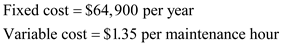1.The variable rate is \$1.35 per maintenance hour. The allocated fixed cost for each using department based on its budgeted peak month usage in maintenance hours is calculated as follows: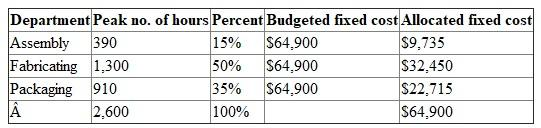In the above table, Percent for the departments are calculated as,2.Assigning the costs of the maintenance department to the user department based on actual usage is as follows: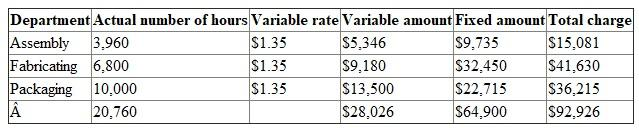3.If the assembly department used 4,000 maintenance hours in the year then the charges would be: